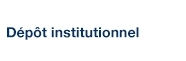# Logarithmic surfaces and hyperbolicity

Dethloff, Gerd et Lu, Steven S.-Y. (2007). « Logarithmic surfaces and hyperbolicity ». Annales de l'Institut Fourier, 57(5), pp. 1575-1610.

Fichier(s) associé(s) à ce document :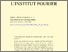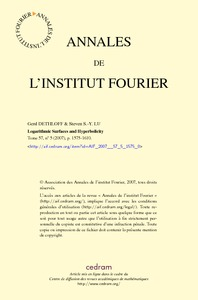Prévisualisation
PDF
Télécharger (616kB)

## Résumé

In 1981 J. Noguchi proved that in a logarithmic algebraic manifold, having logarithmic irregularity strictly bigger than its dimension, any entire curve is algebraically degenerate. In the present paper we are interested in the case of manifolds having logarithmic irregularity equal to its dimension. We restrict our attention to Brody curves, for which we resolve the problem completely in dimension 2: in a logarithmic surface with logarithmic irregularity 2 and logarithmic Kodaira dimension 2, any Brody curve is algebraically degenerate. In the case of logarithmic Kodaira dimension 1, we still get the same result under a very mild condition on the Stein factorization map of the quasi-Albanese map of the log surface, but we show by giving a counter-example that the result is not true any more in general. Finally we prove that a logarithmic surface having logarithmic irregularity 2 admits certain types of algebraically non degenerate entire curves if and only if its logarithmic Kodaira dimension is zero, and we also give a characterization of this case in terms of the quasi-Albanese map.

Type: Article de revue scientifique Classification of logarithmic surfaces; Foliations; Quasi-Albanese Faculté des sciences > Département de mathématiques Steven Lu 09 juin 2016 13:41 27 juin 2016 15:59 http://archipel.uqam.ca/id/eprint/8600

## Statistiques

Voir les statistiques sur cinq ans...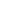RECHERCHER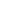PARCOURIR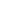LIBRE ACCÈS